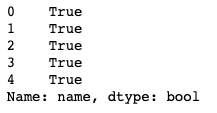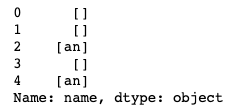Search
•Magdalena Konkiewicz

# 10 Most Useful String functions in pandasImage by ciggy1 from Pixabay

Introduction

If you have been using the pandas library in python you may have noticed that a lot of data comes in textual form instead of pure numbers as some people may imagine.

This means there is a need to clean and preprocess string so it can be analyzed, consumed by algorithms, or shown to the public. Luckily pandas library has its own part that deals with string processing.

In this article, we will walk you through this part of the pandas' library and show you the most useful pandas string processing functions. You will learn how to use:

• upper()

• lower()

• isupper()

• slower()

• isnumeric()

• replace()

• split()

• contains()

• find()

• findall()

Let's get started.

Code set up

In order to demonstrate how our first function works, we should create a pandas data frame that we will be working with. You can use the code below to do it:

```import pandas as pd
client_dictionary = {'name': ['Michael Smith', 'Ana Kors', 'Sean Bill', 'Carl Jonson', 'Bob Evan'],
'grade': [['A', 'A'], ['C'], ['A', 'C', 'B'], [], ['F']],
'age': ['19', '19', '17', '18', '-'],
'group': ['class 1', 'class 2', 'class 2', 'class 1', 'class 2'],
'suspended': [True, False, True, False, True]
}
df = pd.DataFrame(client_dictionary)
df```As a result, you have created a data frame with five columns: name, grade, age, group, and suspended. The columns we will focus on are name, age, and group as those are represented by the string values.

1. upper()

The first function that we will discuss brings all the letters in a string to the upper case. We can apply it to the name column using the following code.

```
df.name.str.upper()

```As you can see the all the letters in names have been changed to upper case.

Note the syntax of the code used to transform the string. You need to first call '.str' before calling the function upper(). The '.str' transforms the series object to its string form on which the actual string operation can be executed.

This will be needed for all string manipulation functions executed on the columns.

2. lower()

Lower() function works similarly to the upper() function but it does exactly the opposite, it lowers all characters in a string. Here you can see the results of calling it on the name column.

```
df.name.str.lower()

```3. isupper()

This function can be called in the same way as upper() or lower(), following '.str' on the column. It will check every string entry in a column if it has all its characters capitalized. Let's call it on the name column again.

```
df.name.str.isupper()

```As you can see this returns a series with all False values. This makes sense as name column has entries with only the first letters of names and surnames capitalized.

Just to see a different behaviour try the following code:

```
df.name.str.upper().str.isupper()

```As you can see now the function isupper() returns a series with only True values now. This is because we have called it on name column that we have capitalized with upper() function beforehand.

4. islower()

This function works the same as isupper() but it checks for the opposite characteristic if all characters are lower case.

You can see that it will return a Series with all Flase values when called on the name column.

```
df.name.str.islower()

```5. isnumeric()

This function checks if the characters in the string are actually digits. All of them have to be digits in order for isnumeric() to return True.

In our data frame, we had age column that we filled in with some strings. Let's call isnumeric() function of age column.

```
df.age.str.isnumeric()

```As you can see we get a Series with True values except for the last entry which is False. If you remember well the original age column in the data frame had all numbers except the last entry which was a '-' (dash).

6. replace()

Another very useful function is replace(). It can be used to replace a part of the string with another one. Let's demonstrate how to use it on the group column. If you remember the group column consisted of 'class 1' and 'class 2' entries.

```
df.group.str.replace('class ', '')

```In the code above we call replace() function with two parameters. The first parameter is the string that needs to be replaced (in our case 'class ') and the second one is what we want to replace it with (in our case it is an empty string). The result of doing this on group column gives us a series with only digits, ('1' and '2').

Note that replace can be also used with regular expressions. What we have done above (removing the 'class ' part) could be done using a regular expression in the following way:

```
df.group.str.replace(r'[a-z]+ ', '')

```We are not going to go into details about regular expressions in python in this tutorial but the code above will replace every alphanumeric word followed by a space with an empty string.

With replace() you can also use case parameter to set the matching to be case sensitive or not.

7. split()

Split() function splits a string on the desired character. It is very useful if you have a sentence and wand to get a list of individual words. You can do that by splitting the string on the empty space (' ').

In our case, we may want to get the name and the surname of the person as individual strings. Note that right now they are listed as one string in 'name' column. This would be done in the following way:

```
df.name.str.split()

```Note that in this case split did not even need a parameter. The default behavior is splitting on the empty space. If you would like to split the string on something else you would have to pass it to split function. For example, the code below illustrated splitting the same column on character 'a'.

```
df.name.str.split('a')

```8. contains()

Contains() function can check if the string contains a particular substring. The function is quite similar to replace() but instead of replacing the string itself it just returns the boolean value True or False.

Let's demonstrate how the function works by calling it on the group column in order to find out if the string contains the number '1':

```
df.group.str.contains('1')

```As you can see the result is the Series of booleans.

Contains() similar to replace() can also be used with case parameter to make it case sensitive or insensitive.

It can also be used with regular expressions. Below you have an example of checking if the string has a numeric character on a group column:

```
df.group.str.contains(r'[0-9]')

```As you can see the result is a Series object with only True values. This is correct as group column contained entries that had either 1's or 2's in its strings.

9. find()

Find() is another function that can be very handy when cleaning your string data. This function will return an index of where a given substring is found in a string.

It is easier to see using an example. Let's try to find out its functionality using the name column. We will search the strings for letter 's'.

```
df.name.str.find('s')

```If you do not remember the name column this is an original data frame.As you can see an entry at index 1 (Ana Kors) has 's' letter as its 7th character. Also, entry at index 3 (Carl Jonson) has 's' as its 8th character. The rest of the entries resulted in '-1' as they do not contain 's' character at all.

Note that if there are multiple 's' characters found find() would return the index of the first one.

10. findall()

Findall() similarly to find() will search a string for existing substring but instead of one index, it will return a list of matching substrings.

Again it is best to see using a real example. Let's search the name column for 'an' substrings.

```
df.name.str.findall('an')

```We can see that there was one occurrence of 'an' at row 1 and also at row 4.

The default behavior of the findall() function is case insensitive. If you want to ignore the case you can do it by using flags parameter and importing re module.

```
import re
df.name.str.findall('an', flags=re.IGNORECASE)

```As you can see now the entry at index 1 also returns 'An'.

Summary

This article has presented my favorite string processing functions from pandas module and YOU made it to the end! Congrats!

I hope you will be now able to use functions from this tutorial to clean up your own data sets.

Happy coding!